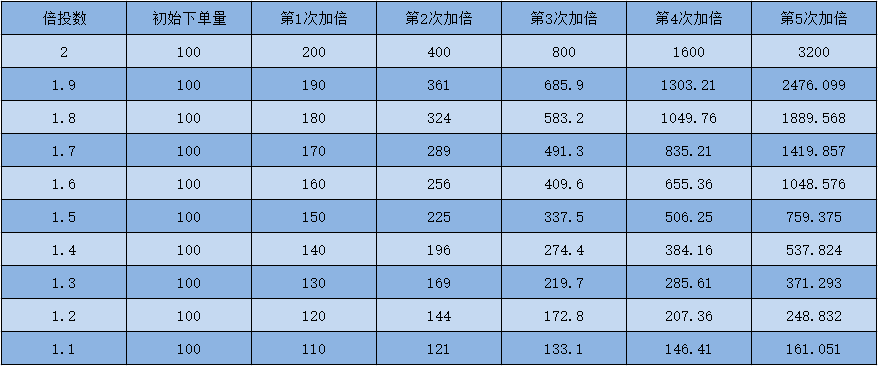# CTA策略之商品期货简易马丁格尔

Author: Zer3192, Date: 2021-04-23 21:06:05
Tags:

#### 三、策略回测

• 回测开始日期：2015-06-01
• 回测结束日期：2021-04-01
• 数据品种：菜粕指数
• 数据周期：日线
• 滑点：开平仓各2跳

#### 四、马丁格尔策略升级#### 五、总结

```/*backtest
start: 2015-06-01 00:00:00
end: 2022-04-01 00:00:00
period: 1d
basePeriod: 1d
exchanges: [{"eid":"Futures_Binance","currency":"BTC_usdt"}]
*/

MarginLevel =20//合约杠杆
unit =0.015//初始下单量
profits =1//盈亏间距
bei =1//倍率

function main() {
exchange.SetContractType("swap")
exchange.SetMarginLevel(MarginLevel)
while (true) {
let depth = exchange.GetDepth();
if (!depth) return;
let bid = depth.Bids.Price==-1;
let position = exchange.GetPosition()
if (position.length == 0) {
let redom = Math.random()
unit =0.015
if (redom > 0.5) {
exchange.SetDirection("sell")
exchange.Sell(-1, unit, "开空")
}
if (redom < 0.5 ) {
}
}
if (position.length > 0) {
let type = position.Type;
let profit = position.Profit;
let amount = position.Amount;
if (type == 0) {
if (profit > profits) {
exchange.Sell(-1, amount, "多头止盈，当前盈利：" + profit)
unit = 0.015
}

if (profit <-profits ) {
unit = unit * bei
}
}

if (type == 1) {
if (profit > profits) {
exchange.SetDirection("closesell")
unit = 0.015
}

if (profit < -profits) {
unit = unit * bei
exchange.SetDirection("sell")
exchange.Sell(-1, unit, "空头加仓，当前盈利：" + profit)
}

}
}

Sleep(1000 )
}
}

```

More